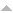## Publications

Export 13 results:
Sort by: Author [ Title] Type Year
O
Fernandes, Vítor H. "On divisors of pseudovarieties generated by some classes of full transformation semigroups." Algebra Colloq.. 15 (2008): 581-588.
Fernandes, Vítor H., and M. V. Volkov. "On divisors of semigroups of order-preserving mappings of a finite chain." Semigroup Forum. 81 (2010): 551-554.Website
Araújo, Isabel M., Mário J. J. Branco, Vitor H. Fernandes, Gracinda M. S. Gomes, and N. Ruškuc. "On generators and relations for unions of semigroups." Semigroup Forum. 63 (2001): 49-62.
Fernandes, Vítor H., M. M. Jesus, and B. Singha. "On orientation-preserving transformations of a chain." Communications in Algebra (DOI 10.1080/00927872.2020.1870996). 49.6 (2021): 2300-2325. AbstractWebsite

In this paper we introduce the notion of an orientation-preserving transformation on an arbitrary chain, as
a natural extension for infinite chains of the well known concept for finite chains introduced in 1998 by McAlister and, independently, in 1999 by Catarino and Higgins.
We consider the monoid POP(X) of all orientation-preserving partial transformations on a finite or infinite chain X and its submonoids OP(X) and POPI(X) of all orientation-preserving full transformations and of all orientation-preserving partial permutations on X, respectively.
The monoid PO(X) of all order-preserving partial transformations on X and its injective counterpart POI(X) are also considered.
We study the regularity and give descriptions of the Green's relations of the monoids POP(X), PO(X), OP(X), POPI(X) and POI(X).

Fernandes, Vítor H., Preeyanuch Honyam, Teresa M. Quinteiro, and Boorapa Singha. "On semigroups of endomorphisms of a chain with restricted range." Semigroup Forum (DOI: 10.1007/s00233-013-9548-x). 89.1 (2014): 77-104. AbstractWebsite

Let $X$ be a finite or infinite chain and let $\O(X)$ be the monoid of all endomorphisms of $X$.
In this paper, we describe the largest regular subsemigroup of $\O(X)$ and Green's relations on $\O(X)$.
In fact, more generally, if $Y$ is a nonempty subset of $X$ and $\O(X,Y)$ is the subsemigroup of $\O(X)$ of all elements with range contained in $Y$,
we characterize the largest regular subsemigroup of $\O(X,Y)$ and Green's relations on $\O(X,Y)$.
Moreover, for finite chains, we determine when two semigroups of the type $\O(X,Y)$ are isomorphic and calculate their ranks.

Fernandes, Vítor H., Preeyanuch Honyam, Teresa M. Quinteiro, and Boorapa Singha. "On semigroups of orientation-preserving transformations with restricted range." Communications in Algebra (DOI:10.1080/00927872.2014.975345). 44.1 (2016): 253-264. Abstractauthorsfinalversion.pdfWebsite

Let $X_n$ be a chain with n elements ($n\in\N$) and let $\OP_n$ be the monoid of all orientation-preserving transformations of $X_n$. In this paper, for any nonempty subset $Y$ of $X_n$, we consider the subsemigroup $\OP_n(Y)$ of $\OP_n$ of all transformations with range contained in $Y$: we describe the largest regular subsemigroup of $\OP_n(Y)$, which actually coincides with its subset of all regular elements, and Green's relations on $\OP_n(Y)$. Also, we determine when two semigroups of the type $\OP_n(Y)$ are isomorphic and calculate their ranks. Moreover, a parallel study is presented for the correspondent subsemigroups of the monoid $\OR_n$ of all either orientation-preserving or orientation-reversing transformations of $X_n$.

Delgado, Manuel, V{\'ı}tor H. Fernandes, Stuart Margolis, and Benjamin Steinberg. "On semigroups whose idempotent-generated subsemigroup is aperiodic." Internat. J. Algebra Comput.. 14 (2004): 655-665.Website
Fernandes, Vítor H., and Tânia Paulista. "On the monoid of partial isometries of a finite star graph." Communications in Algebra (DOI 10.1080/00927872.2022.2121404; Online 14 Sep 2022). 51.3 (2023): 1028-1048. AbstractWebsite

In this paper we consider the monoid DPSn of all partial isometries of a star graph Sn with n vertices. Our main objectives are to determine the rank and to exhibit a presentation of DPSn. We also describe Green’s relations of DPSn and calculate its cardinal.

Fernandes, Vítor H., and Teresa M. Quinteiro. "On the monoids of transformations that preserve the order and a uniform partition." Communications in Algebra. 39.8 (2011): 2798-2815.
Fernandes, Vítor H., and Tânia Paulista. "On the Rank of Monoids of Endomorphisms of a Finite Directed Path." Asian-European Journal of Mathematics (DOI 10.1142/S1793557123500699; Online 28 Oct 2022). 16.04 (2023): 2350069 (13 pages). AbstractWebsite

In this paper we consider endomorphisms of a finite directed path from monoid generators perspective. Our main aim is to determine the rank of the monoid wEndP_n of all weak endomorphisms of a directed path with n vertices, which is a submonoid of the widely studied monoid O_n of all order-preserving transformations of a n-chain. Also, we describe the regular elements of wEndP_n and calculate its size and number of idempotents.

Fernandes, Vítor H., and Jintana Sanwong. "On the rank of semigroups of transformations on a finite set with restricted range." Algebra Colloquium. 21.3 (2014): 497-510.authorsfinalversion.pdfWebsite
Fernandes, Vítor H., and Teresa M. Quinteiro. "On the ranks of certain monoids of transformations that preserve a uniform partition." Communications in Algebra. 42.2 (2014): 615-636.
Fernandes, Vítor H. "Oriented transformations on a finite chain: another description." Commun. Korean Math. Soc. (In Press). Abstract

Following the new description of an oriented full transformation on a finite chain given recently by Higgins and Vernitsk,
in this short note we present a refinement of this description which is extendable to partial transformations and to injective partial transformations.